### Course Description

• This course is sign on statistics.
• It will told us some models, and explain them station.
• like regression, ANOVA, ANCOVA, and so on

### key point before test

1、作业1的第1题，第1-2章的课件。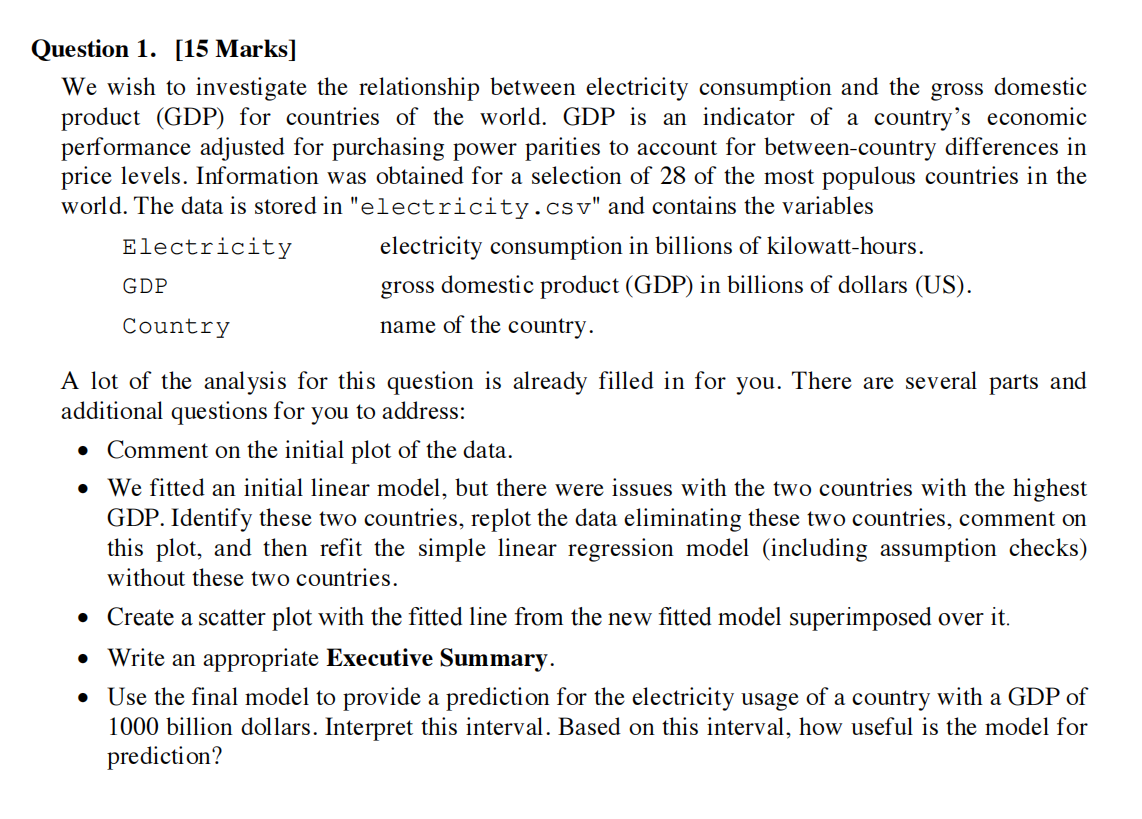• Look at the question：

• Goal: Inverstigate the relationship between electricity consumption and GDP.

• Data: A file named electricity.csv, coverd Electricity, GDP, Country name

• T1: Comment the initial plot of the data

• word notes:

• If we want to comment the initial plot of the data, we need get this plot before.

``````elec.df <- read.csv("electricity.csv")
plot(Electricity~GDP, data=elec.df)
# show the data plot, and entitled it
``````
• and the outcome is here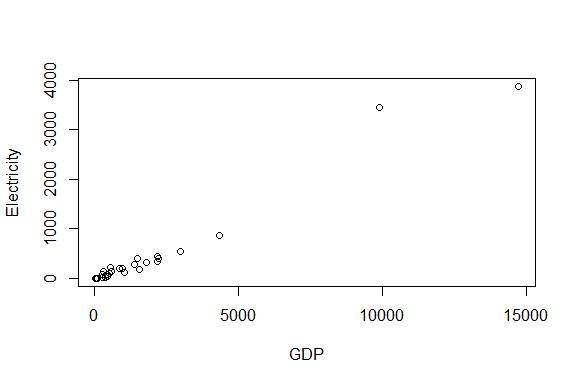In the plot, when GDP less than 5000, It is clearly that there are some relationship between GDP and Electricity.

the Electricity will increased by growth with GDP.

• T2: We fitted an initial linear model, but there were issues with the two countries with the highest GDP. Identify these two countries, replot the data eliminating these two countries, comment on this plot, and then refit the simple linear regression model (including assumption checks) without these two countries.

• word notes:

• First, calculate the residuals and fitted valued.

``````elecfit1=lm(Electricity~GDP,data=elec.df)
# function lm: calculate the residuals and fitted value.
plot(elecfit1,which=1)
# show the plot
word notes:{calculate:计算, residuals:残差, fitted value: 拟合值}
``````
• The outcome is here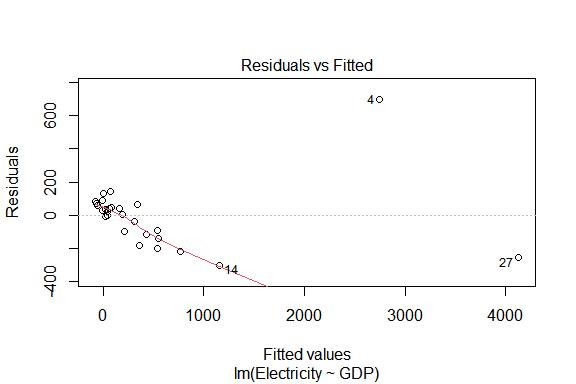• Then, get the norm check to this data

``````normcheck(elecfit1)
``````
• The outcome in here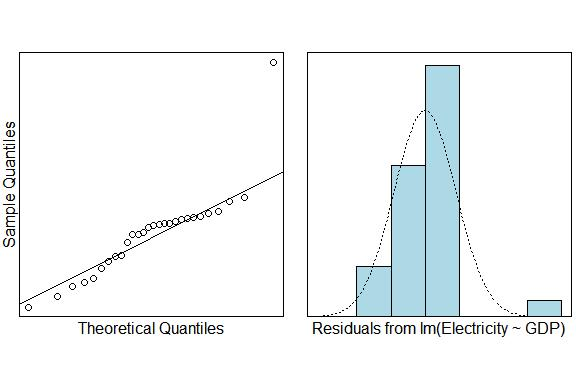• Then, get the Cook`s distance plot

``````cooks20x(elecfit1)
``````
• The outcome in here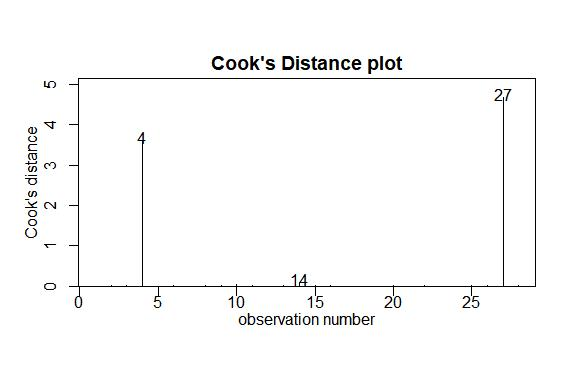• In the cooks distance plot, there are two data is far from others. Its the 4th and 27th. It have a disproportionate impact to the model. And, according to residuals and fitted valued, we can infer that those two countries is which GDP more than 5000.

• now, del those data and replot data.

``````data=elec.df[elec.df\$GDP<5000,]
# del data which GDP over 5000
plot(Electricity~GDP,data)
# and show the plot
``````
• Okay, now we only have the last part: check again

``````elecfit2=lm(Electricity~GDP,data=elec.df[elec.df\$GDP<6000,])
plot(elecfit2,which=1)
# get data, and show original plot

normcheck(elecfit2)
# norm check

cooks20x(elecfit2)
# cooks distance
``````
• T3 Create a scatter plot with the fitted line from the new fitted model superimposed over it.

• word notes:

``````plot(Electricity~GDP,data=elec.df[elec.df\$GDP<6000,])
# get plot
elecgdp.fit=lm(Electricity~GDP,data=elec.df[elec.df\$GDP<6000,])
abline(elecgdp.fit,lty=2,col="red")
``````
• T4 Write an appropriate Executive Summary.

• word notes:
• We wish to investigate the relationship between electricity consumption and the gross domestic product (GDP) for countries of the world. First,we read in and inspect the data and get an initial plot. Then,we use lm() to fit a appropriate linear model and use normcheck() and cooks20x() to do model checks. To make estimation credible,we eliminate two countries with GDP greater than 6000. Because their Cook’s distances are greater than 0.4. Then,we replot data and fit a more appropriate linear model by same way. Finally,we create a scatter plot with the fitted line from your model superimposed over it.
• word notes:
• We want to find a relationship between the GDP and the electricity consumption. First, we read the data from file and get an initial plot. And then, we use the function lm to fit a appropriate linear model and use two function named normcheck and cooks20x to do model checks. We find two countries have different data, because the cooks distance is more than 0.4. So, we eliminate them, and get initial plot again, and do model check again. Finally, we create a scatter plot with the fitted line from model superimposed over it.

2、会对x-y的散点图进行评论。（课件第1章第20页）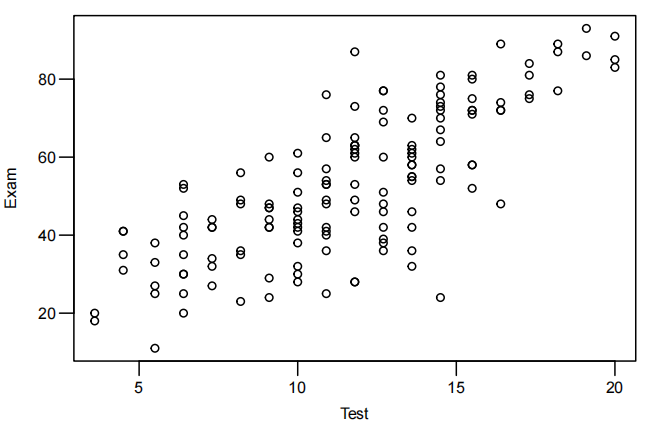• Step one: some fixed describtion

Looking at this plot, it is clear that there is some relationship.

but there is also a lot of variability in exam score amongst students with the same test score, especially in the middle of the data.

3、会对同一数据应用不同模型进行拟合的图进行评论。（课件第2章第,31页）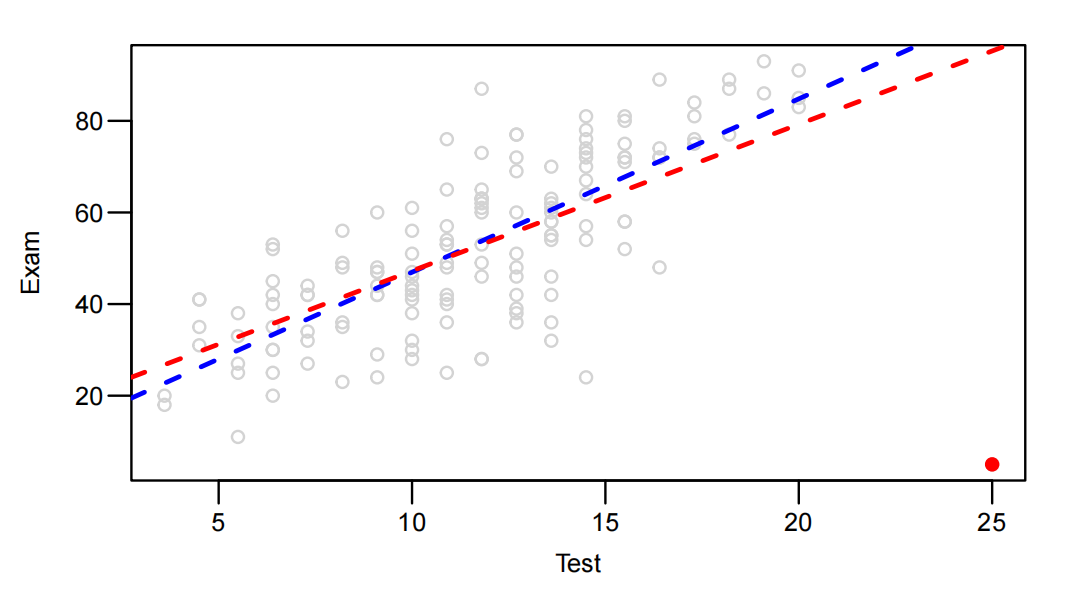• First, judge the type of two models(linear), describe the positive/negative, the slope of them.

• Next, Prediction results: When is it close, when is it far away, and why? As can be seen in the figure, the two lines are close to each other in which range between x, and far away in which range?.

• The reasons for this phenomenon are; the existence of points; The middle block has more data, while the two sides have less data. When the amount of data is large, different models have different resistance to outliers

• Like:

• They are two positive linear model, at first, the scope of red one is more than the blue one, and then end, the station is opposite.
• Those two line is closed at middle, and be far at two side. I guess the reason is, the point almost focus on the middle, only few on the side.

4、知道summary这个函数输出结果中每个数字的含义。（课件第2章第40-46页）

• This part only about translate.
• word notes:
• Estimate 估计值
• Std. 标准差
• Error 误差
• Intercept 截距
• Slope 斜率
• Residual standard error 残差标准误差
•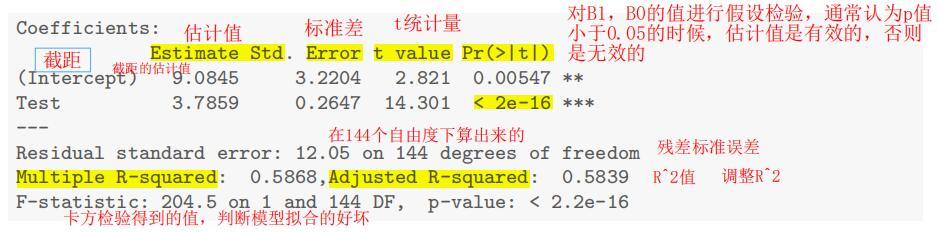5、会结合程序描述E(y)的置信区间（CI）和y的预测区间（PI）的具体含义。（课件第2章第48-50页）

• If the code is:

``````preds.df=data.frame(Test=c(0,10,20))
predict(examtest.fit, preds.df)
``````
• and the outcome is:

``````1 2 3
9.084463 46.943703 84.802942
``````
• These values are our estimates of the expected Exam scores for students with Test scores of 0, 10 or 20, respectively.

• If we want to get the CI, then use those code:

``````predict(examtest.fit, preds.df, interval="confidence")
``````
• The outcome is:

``````fit lwr upr
1 9.084463 2.71902 15.44991
2 46.943703 44.80912 49.07828
3 84.802942 79.97021 89.63568
``````
• Then, we know that, the students who is grade 0, the average forecast ranged from 2.7 to 15.4.

Then, we know that, the students who is grade 10, the average forecast ranged from 46.9 to 49.1.

Then, we know that, the students who is grade 20, the average forecast ranged from 80.0 to 90.0.

Need keep one decimols!

• If we wawnt to get the PI, then use those code:

``````predict(examtest.fit, preds.df, interval="prediction")
``````
• The outcome is:

``````fit lwr upr
1 9.084463 -15.56475 33.73368
2 46.943703 23.03510 70.85231
3 84.802942 60.50438 109.10151
``````
• And we know:

The 95% prediction interval of the students who in grade 0 is -15.6 to 33.7

and so on

6、Methods and Assumption Checks的写法。（参考作业1的第1题）

• A.1 Method and Assumption Checks
• A.1.1 核心内容：用专业术语对建模过程进行全面叙述。
• A.1.2 叙述我们要研究的问题是什么。（问题描述）
• 解析：这个问题的描述可以从题中直接得到。
• 案例：我们要研究 test 与 exam 分数之间的关联关系。
• A.1.3 叙述我们为什么采用这种模型进行建模。（观察数据）
• 解析：我们可以从数据呈现出的分布规律进行总结说明，也可以从数据类型中进行说明，
也可以从经验常识的角度进行说明。
• 案例：因为 x 与 y 的数据呈现线性分布规律，因此我们选择线性模型进行拟合分析。
• A.1.4 叙述我们是否需要对模型进行调整。（建模过程）
• 解析：模型的残差图、QQ 图、Cook 距离等，是否支持我们使用当前模型。模型中是否
需要引入新的自变量或去掉部分自变量。模型中的自变量及因变量是否需要进行额外的变换
操作。
• 案例：我们一开始使用的是简单线性模型，但是从残差图上看，数据呈现分散分布趋势，
因此我们在简单线性模型的基础上添加 x 的二次项后残差图呈现带状分布趋势，满足了线
性模型的使用条件。
• A.1.5 叙述模型具体形式（模型表示）
• 解析：需要将模型的具体形式和所有变量的含义完整表述清楚，不能漏项。
• 案例：𝑦𝑦𝑖𝑖 = 𝛽𝛽0 + 𝛽𝛽1𝑥𝑥𝑖𝑖 + 𝜀𝜀𝑖𝑖，𝜀𝜀𝑖𝑖
中𝑥𝑥𝑖𝑖表示 test 的成绩，𝑦𝑦𝑖𝑖表示 exam 的成绩。
• A.1.6 叙述模型评价指标（模型评价）
• 解析：通过𝑅𝑅2对模型拟合数据的好坏进行说明。
• 案例：我们的模型解释了的期末考试成绩中 59%的数据（变量）
• A.2 Executive Summary
• A.2.1 核心内容：用一般术语对建模结果进行全面总结。
• A.2.2 说明我们要研究的问题是什么，并回答此问题。（结论简述）
• 解析：这个问题的描述可以从题中直接得到。通过前文的分析给出结论。
• 案例：我们要研究 test 与 exam 分数之间的关联关系。test 与 exam 之间存在线性关系。
• A.2.3 说明我们得出上述结论的依据是什么。（证据说明）
• 解析：先说明有没有证据支持这一结论，再说明证据的是啥。
• 案例：我们有很强的证据表明 test 与 exam 分数之间存在线性关系，因为 p 值小于 0.05。
• A.2.4 对结论进行定量说明。（结论详述）
• 解析：需要对我们研究的问题进行定量叙述。这种叙述需要结合具体数据。
• 案例：test 分数每增加 1 分，exam 的平均分数就会增加 3.3 到 4.3 分之间。
• A.2.5 对预测值进行定量说明。（预测详述）
• 解析：如果题目中要求进行预测，我们需要对预测结果进行说明。
• 案例：test 分数为 10 分的那些人，exam 的平均分数在 44.8 到 49.1 分之间。
案例：test 分数为 10 的张三，他 exam 的分数预计在 23.0 到 70.9 分之间。

1、第6章课件，第6章案例。
2、应用乘法模型对数据拟合的三个原则。（课堂笔记L06-1）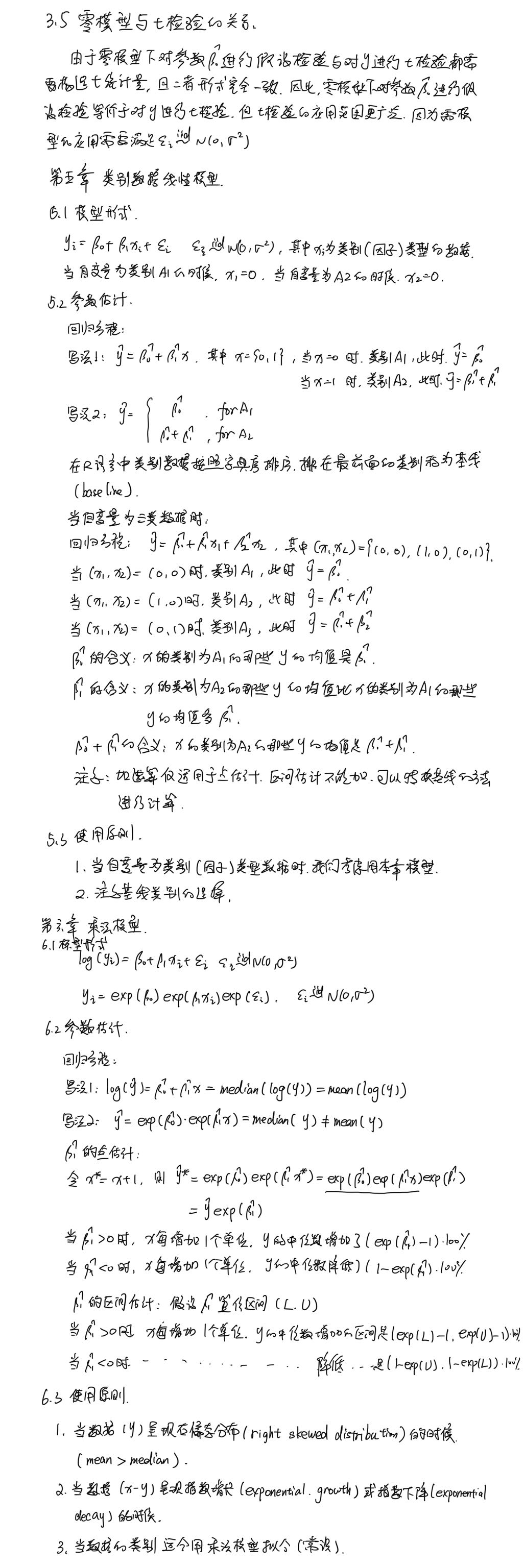3、乘法模型的形式。（第6章案例）

• 直接看第六章案例

4、Executive Summary的写法。（第6章案例）

• 直接看第六章案例

## 111

Data AnalysisCourse DescriptionThis course is sign on statistics.It will told us some models, and explain them station.like regression, ANOVA, ANCOVA,…